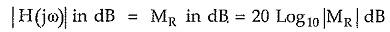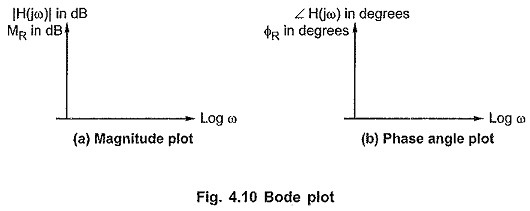## Bode Plot in Network Analysis:

The Bode Plot in Network Analysis is a popular method to obtain the frequency response of the networks. It basically sketches the variations in MR and ΦR against the frequency ω but uses a logarithmic scale for ω, to accommodate large range of frequency within a small distance. Similar to the frequency response, the Bode plot also consists of two plots,

1. Magnitude plot
2. Phase angle plot

### Magnitude Plot:

The scientist Bode suggested to express MR in decibel (dB) to plot against Log (ω). Such a dB value of MR can be obtained as,Such dB values are obtained for various values of ω from 0 to ∞ and are plotted against Log10 ω.

The magnitude plot is shown in the Fig. 4.10 (a).### Phase Angle Plot:

The ∠ H(jω) i.e. ΦR is obtained in degrees for various values of ω and are plotted against Log10 ω.

The phase angle plot is shown in the Fig. 4.10 (b).

Scroll to Top